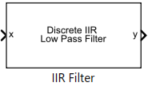# IIR Filter

Implement infinite impulse response (IIR) filter

• Library:
• Motor Control Blockset / Signal Management

•## Description

The IIR Filter block implements a discrete infinite impulse response (IIR) low pass filter on the specified input signal. The block supports fixed point and floating point data types.

### Equations

The block uses this equation to implement the IIR filter:

`$y\left(k\right)=a×{x}_{k}+\left(1-a\right)×{y}_{k-1}$`

where:

• $a$ is the filter coefficient in the range (0, 1].

• $y\left(k\right)$ is the filtered output value at time $k$.

• ${y}_{k-1}$ is the filtered output value at time $k-1$.

• ${x}_{k}$ is the sampled input value at time $k$.

You can define a filter coefficient in the block parameter. A lower filter coefficient gives better filtering, but it increases the delay in the response time.

## Ports

### Input

expand all

Sampled values of the raw input signal in the time domain.

Data Types: `single` | `double` | `fixed point`

### Output

expand all

Filtered output signal returned by the IIR Filter block in the time domain.

Data Types: `single` | `double` | `fixed point`

## Parameters

expand all

Filter coefficient of the IIR block.

Get ebook